# Polynomial. Division of polynomial by polynomial

Definition: a Polynomial of one variableis a polynomial of the form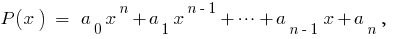where thenumerical coefficients.

Definition: Ifthis polynomial is called the polynomial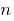of the first degree relatively variable.

Membercalled a senior member of the polynomial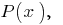a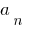— it free member.— the polynomial of the third degree.

## Identically equal polynomials in one variable

Definition: Two polynomials are called equalif they take equal values for all values of the variable.

## Properties identical with equality of polynomials in one variable

1. If the polynomial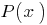is identically equal to zero (i.e. has zero values at all values), then all of its coefficients are equal to zero.
2.3. If two polynomial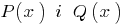identically equal to (i.e., acquire the same value at all values), then they coincide (i.e., their degrees are equal and the coefficients of equal powers of equal).

## Division of polynomial by polynomial

Definition: If two polynomials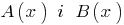it is possible to find a polynomial ,thatis divided into.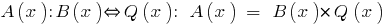### Example

Since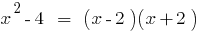, the polynomial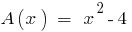is divisible by the polynomial## The division of the polynomial by the polynomial s Stacey

Definition: a Polynomialis divided by the polynomials Stacey, if you can find a pair of polynomials,and the degree of the remainder of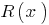smaller degree.

If the remainder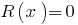, then the polynomialis divisible by the polynomialwithout a remainder)

### Example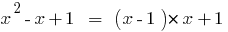,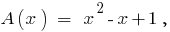## The division of the polynomial by the polynomial "area"

### The rule of division of polynomials in one variable

1. To place the members of the polynomials with descending exponents of the variable.
2. To share a senior member of the dividend on the senior member of the divider.
3. The result is multiplied by the divisor and subtract this product from the dividend.
4. With the obtained difference perform the same operation: divide it senior member the senior member of the divider and the result again multiplied by the divisor, and so on. This process continue to give until I get the balance to zero (if one polynomial divided by another) or as long as the balance does not get the polynomial degree is less than degree of divisor.

## Theorem Continuous

The remainder of dividing the polynomialon doclenequal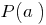Corollary: If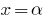is a root of the polynomial(i.e.,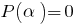), then this polynomial is divided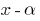.

### Example

The remainder of dividing the polynomialon doclen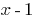equal, that isdivided intowithout a remainder.

Dividinginto"area" or Horner's scheme, we get: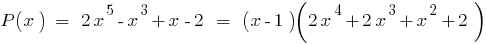Tags:
Chapter:
Versions in other languages:
Share with friends: